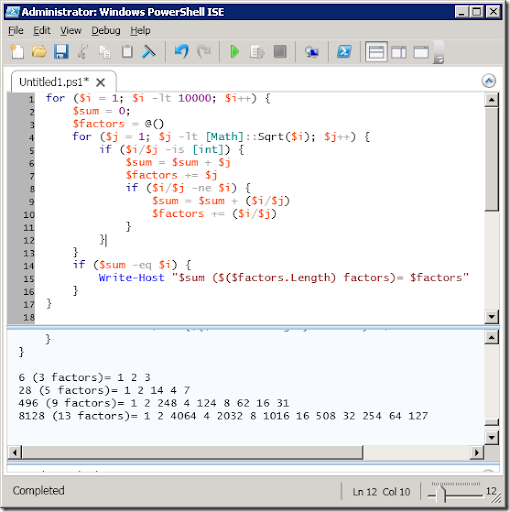My 10 year old daughter came home from school today with a simple math problem that she had to solve and she asked if there was an easier way to solve the problem. Basically the teacher explained that the number six was a perfect number because the sum of it’s factors (excluding itself) is equal to the number and she wanted to know what the next perfect number was. The kids basically had to go through the numbers and manually add up the factors until they found a match. So naturally, the programmer in me said, sure we can write a quick algorithm to find the next few perfect numbers.

After writing the code, using PowerShell of course, I realized that this little math algorithm demonstrated a few key PowerShell concepts that would be good for anyone starting out with PowerShell to know. Here’s the code I wrote for her along with the output:So in this really simple math example you can see how to use a basic for loop construct, dynamic arrays, dynamic typing, static method calls, and variable replacement within strings.

As you can see, for loops in PowerShell are identical to those in C# so there’s nothing new there (unless you’re new to C# that is). Dynamic arrays on the other hand are kind of cool – you can declare an empty array by simply using `@()`. Don’t confuse this with declaring empty hash tables which use curly braces instead of parenthesis, `@{}`. Adding elements to these arrays is as easy as using the `+=` operator: `\$factors += \$i`.

You can see the dynamic typing where I’m dividing `\$i` by `\$j` – if it divides evenly then the type returned would be an integer, otherwise it would be a float. So a really easy way to check if it divided evenly is to see if the returned type is equal to `[int]` (we can work with a type by wrapping a type name in brackets).

Calling static methods is a little different than what you may be used to in C#. As mentioned above types are defined by wrapping the type name in brackets – if we want to call a static method (or access a static property) of a type then we simply separate the method or property name and the type name with double colons. So in this example I’m finding the square root of the number by using the static `Sqrt` method on the `System.Math` class: `[Math]::Sqrt(\$i)`.

The last little bit, dynamic variable replacement, just demonstrates how we can use `\$()` to force the contents of the parentheses to be evaluated before they are used within the string for the Write-Host command. In this case I wanted to show the number of factors but if I did not wrap the `\$factors.Length` bit in parentheses my output would look like this: `6 (1 2 3.Length factors)= 1 2 3`.

So, as you can see, PowerShell is a really great tool and can be used for things other than SharePoint, even helping your 4th grader with her math homework 🙂